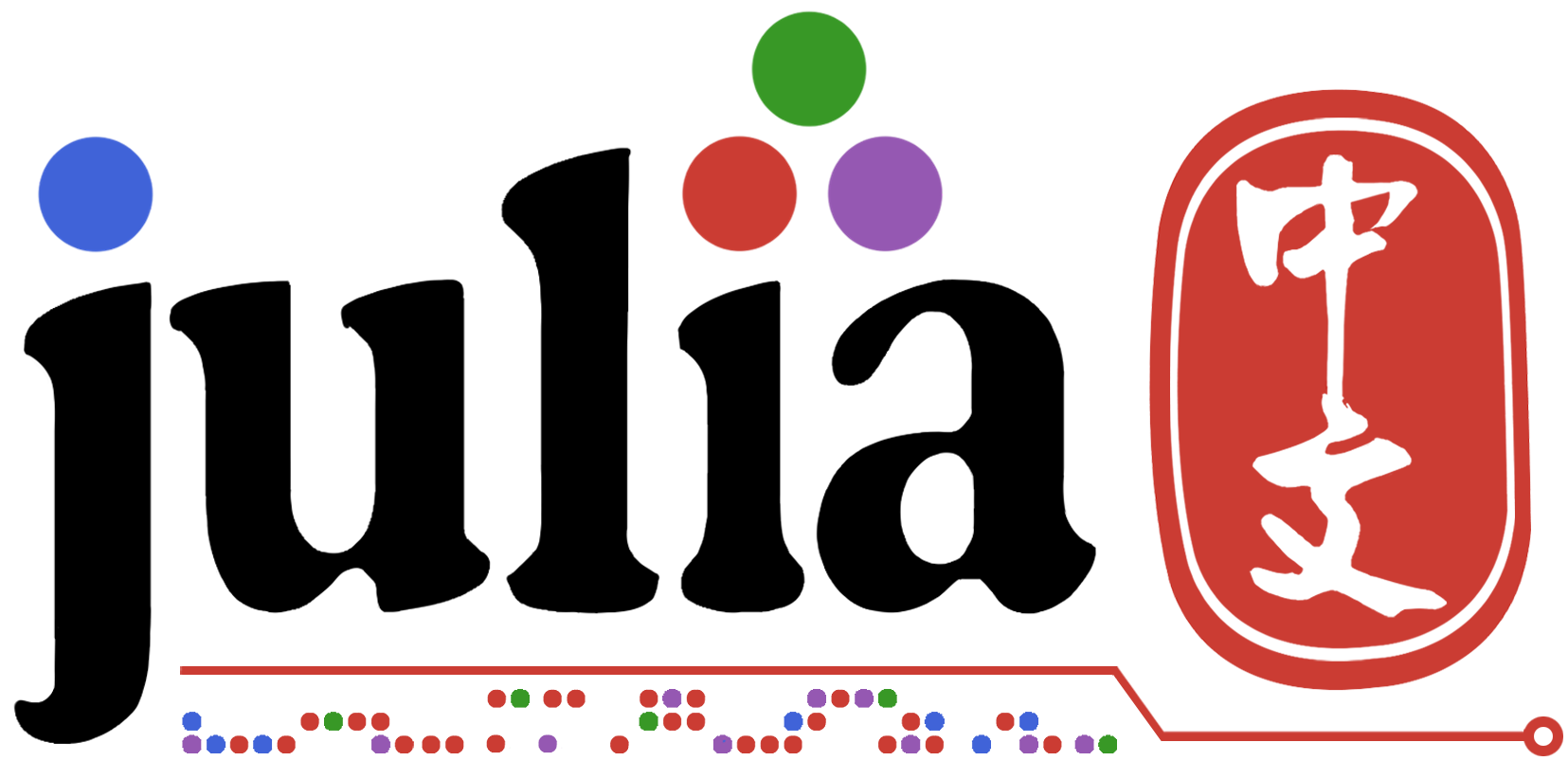# 今天先从简单的线性回归入门

h\theta(x)=\theta0+\theta1x的情况还不会，毕竟能力有限

\theta1 :=\theta1- \alpha * J(\theta)(这里导数不会打)直到导数接近0

import CSV
using DataFrames
using Plots

list=[x for x in -50:50]#这个list用来辅助下面的symbollist
symbollist=[Symbol(:D,x) for x in list]#用来命名df中的列名

for θ1 in -50:50
df[!,symbollist[θ1+51]] = df[!,:L2] * θ1 #hθ(x) = θ1*x
end

list=Vector{Float64}(undef,101)#名字一样，不过无所谓啦,这里用作代价函数

for i in -50:50
list[i+51] = sum((df[!,symbollist[i+51]] - df[!,:L2]).^2)/50
end

scatter(-50:1:50,list)


L1,L2
1,1.1
1,0.9
1,1.0
2,2.1
2,2.2
2,1.95
2,1.99
3,2.9
3,2.89
3,2.97
3,2.9
4,3.8
4,4.1
4,4.2
4,3.98
4,3.99
5,4.988
5,4.97
5,5.001
5,5.0
5,5.005
6,6.001
6,5.999
6,6.002
6,5.899

dθ0, dθ1 = grad(f, x)
θ0 = θ0 - a*dθ0
θ1 = θ1 - a*dθ1


df[!,symbollist[θ1+51]] = df[!,:L2]